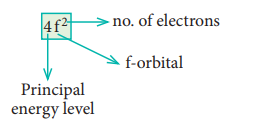Home | | Chemistry 11th std | Evaluate Yourself: Chemistry: Quantum Mechanical Model of Atom

# Evaluate Yourself: Chemistry: Quantum Mechanical Model of Atom

Chemistry : Quantum Mechanical Model of Atom

Evaluate Yourself

1. Calculate the de-Broglie wavelength of an electron that has been accelerated from rest through a potential difference of 1 keV.

Solution:

Given: accelerated potential = 1 keV

The kinetic energy of the electron = the energy due to accelerating potential.m = mass of the electron = 9.1 ├Ś 10ŌĆō31 kg

h = Planck constant = 6.626 ├Ś 10ŌĆō34 Js 1

1eV = 1.6 ├Ś 10ŌĆō19 J2. Calculate the uncertainty in the position of an electron, if the uncertainty in its velocity is 5.7 ├Ś 105 ms-1.

Solution:3. How many orbitals are possible in the 4th energy level? (n=4)

Solution:

n = 4

l = 0, 1, 2, 3

Ōł┤ 4 sub shells s, p, d & f.

l = 0 ml = 0 ŌćÆ one 4s orbital.

l = 1 ml = ŌĆō1, 0, + 1 ŌćÆ three 4p orbitals.

l = 2 ml = ŌĆō2, ŌĆō1, 0, +1, +2 ŌćÆ five 4d orbitals.

l = 3 ml = ŌĆō3, ŌĆō2, ŌĆō1, 0, +1, +2, +3ŌćÆ seven 4f orbitals.

Over all 16 orbitals are possible.

4. Calculate the total number of angular nodes and radial nodes present in 3d and 4f orbitals

Solution:5. Energy of an electron in hydrogen atom in ground state is -13.6 eV. What is the energy of the electron in the second excited state?

Solution:6. How many unpaired electrons are present in the ground state of Fe3+ (z=26), Mn2+ (z=25) and argon (z=18)?

Solution:Electronic configuration of Mn2+ is 1s2 2s2 2p6 3s2 3p6 4s0 3d5

Five unpaired electrons.

Electronic configuration of Ar: 1s2 2s2 2p6 3s2 3p6

no unpaired electrons.

7. Explain the meaning of the symbol 4f2. Write all the four quantum numbers for these electrons.

Solution:n = 4 ; f orbital l = 3 ŌćÆ ml = ŌĆō 3, ŌĆō2, ŌĆō1, 0, +1, +2

Out of two electrons, one electron occupies 4f orbital with ml = ŌĆō3 and another electron occupies 4f orbital with ml = ŌĆō2.

All the four quantum numbers for the two electrons are8. Which has the stable electronic configuration? Ni2+ or Fe3+.

Solution:

Electronic configuration of Fe3+ : 1s2 2s2 2p6 3s2 3p6 4s0 3d5

Electronic configuration of Ni2+ : 1s2 2s2 2p6 3s2 3p6 4s0 3d8

Fe3+ has stable 3d5 half filled configuration.

Tags : Solved Example Problems , 11th Chemistry : UNIT 2 : Quantum Mechanical Model of Atom
Study Material, Lecturing Notes, Assignment, Reference, Wiki description explanation, brief detail
11th Chemistry : UNIT 2 : Quantum Mechanical Model of Atom : Evaluate Yourself: Chemistry: Quantum Mechanical Model of Atom | Solved Example Problems